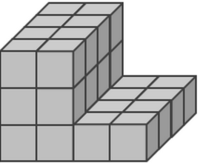100

36x9=

324

100

What are the formulas for volume?

V = (l x w) x h

100

3 oranges are cut into halves. How many people can have 1/2 of an orange?

6 people

100

What part of a division problem is the answer?

quotient

100
True or false: 432 x 12 = 5,184

True

200

324 x 6=

1,944

200

A square has a length of 4/5 inch and width of 2/3 inch. What is the AREA of the square?

8/15 square inches

200

5/7 x 3/6

15/42

200

96 divide 8

12
200

True or False: 6,843 x 5 = 34,210

False

300

8,251 x 4=

33,004

300

A square has one side that is 12 cm long.  What is the AREA of the square?

144 square cm

300

3 x 4/5

12/5 = 2 2/5

300

134 divide 6 =

22 R 2

300

What do you call an answer to a multiplication equation.

The Product.

400

13 X 85=

1,105

400

What is the volume?100 cubic cm

400

3 ÷ 1/2

6

400

1,243 divide 4 =

310 R 3

400

4,027-2,738=?

1,289

500

1,356 x 12 =

16,272

500

What is the volume?32 cubic units

500

5 friends share 1/2 pan of mac and cheese. How much does each friend get?

1/10 pan

500

1,528 divide 5 =

305 R 3

500

How many minutes are in 1 hour and 25 minutes?

85 minutes

Click to zoom